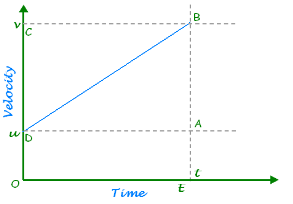# Derive an equation for velocity time relation by graphical method

Derive an equation for velocity time relation by graphical method

Let an object is moving with uniform acceleration.Let an object is moving with uniform acceleration.

Let the initial velocity of the object = u
Let the object is moving with uniform acceleration, a.
Let object reaches at point B after time, t and its final velocity becomes, v
Draw a line parallel to x-axis DA from point, D from where object starts moving.
Draw another line BA from point B parallel to y-axis which meets at E at y-axis.
Let OE = time, t
Now, from the graph,
BE = AB + AE
⇒ v = DC + OD (Since, AB = DC and AE = OD)
⇒ v = DC + u (Since, OD = u)
⇒ v = DC + u ------------------- (i)
Now, Acceleration (a) =(Change in velocity)/(Time taken)

a= v – ut
⇒ a = v - ut
⇒ a= OC – OD t = DC t
⇒a = OC-OD t =DC t
⇒at=DC
⇒at=DC -----(ii)
By substituting the value of DC from (ii) in (i) we get
v= at + u
v = at + u
⇒ v = u + at
⇒ v = u + at
Above equation is the relation among initial velocity (u), final velocity (v), acceleration (a) and time (t). It is called first equation of motion.